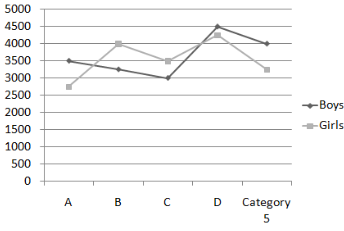Open In App

# Quantitative Reasoning in GRE General

The Quantitative Reasoning Section is the most scoring section of GRE. This section evaluates the aspirants on their basic mathematical concepts of algebra and geometry. For computer-based GRE, an onscreen calculator is provided to the aspirants and for paper-based GRE, the test centre provides calculator to the aspirants. The problems of this section may be set in real or mathematical situations. Few questions of this section may be combined and collectively are answered based on interpretation of the data or table provided. Such questions are called data interpretation type questions. This section includes four types of questions:

1. Quantitative Comparison Type Questions
2. Multiple Choice Type Questions
3. Multiple Correct Type Questions
4. Numeric Entry Type Questions

Basic mathematical reasoning concepts and logics are tested in this section. Speed and accuracy are the prime necessity for success in result. This section checks the aspirants on the following topics:

1. Arithmetic:
Numbers and their properties, number patterns, representation of number set, operations in arithmetic.

• Example:
What will be the HCF of 436 and 327?

2. Algebra:
Simplification of algebraic expressions, solving simple equality and inequality problems, factorisation, co-ordinate geometry, relations and functions.

• Example:
Vaibhav makes a profit of Rs. 2 per book by selling his old book, but he pays a monthly rent of Rs. 4 per month to the shopkeeper, who takes his books. How many books should he sell so that at least a profit of Rs. 120 per month is made?

```(A) Rs. 62
(B) Rs. 64
(C) Rs. 65
(D) Rs. 66 ```

3. Geometry:
Perimeter, area, volume, congruence and similarity of figures, lines and angles, triangle, quadrilateral and other polygons.

• Example:
If in a triangle RST, RS = RT, ?R = x + 15°, ?S = 2x + 25° then value of T ?

```(A) 64°
(B) 71°
(C) 69°
(D) 78° ```

4. Data Analysis:
Interpretation of data, tables, graphs, charts, pie-charts, Venn-diagrams, random variables, frequency distribution and probability distribution.

• Example:Que-1: What percentage of the total girls are in school A?

```(A) 18
(B) 22
(C) 17
(D) 15 ```

```(A) D